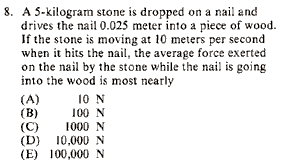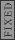GR 8677927796770177 | # Login | Register

GR8677 #8
Problem
 GREPhysics.NET Official Solution Alternate SolutionsMechanics}Conservation

Straight-forward conservation of energy problem:

where , , and . Plug everything in, and solve for , to get choice D, .Alternate Solutions
 casseverhart132019-09-13 07:54:17 Simply desire to say your problem is amazing. click hereReply to this commenttimfinnigan2018-05-19 03:40:44 Simple Work-KE Thm:\r\n\r\n\r\n\r\n\r\n\r\n\r\n\r\nThe magnitude of the force, , is the answer (the negative could be thought of as direction).Reply to this commentdcn2008-03-26 09:21:36 Correct me if I'm wrong but isn't Average Force defined simply as delta p/delta t. Since the stone comes to rest, assuming all momentum is transfered to the nail, the momentum of the stone just before it hits the nail is delta p. For delta t we can use the average v which is (10-0)/2 = 5m/s. Then delta t = y/(average v) =0.025/5. Plugging this in: Average F = (5kg)(10m/s)(5m/s)/(25x10^-3m)=10^4N.Reply to this commentjcain62005-12-09 05:12:15 you can also use kinematics here. Use the velocity/position kinematic to solve for a: a = 2000 m/s^2 where you use 0.025m as change in y. F = ma = (5kg)(200m/s^2)= 10000NReply to this commentcasseverhart13
2019-09-13 07:54:17
Simply desire to say your problem is amazing. click hereernest21
2019-08-10 03:09:53
I see the answers but I cannot find the questions. Where are they? There was a \"jump into the question\" buton in the old site that I can\'t see now. pixelmon islandjoshuaprice153
2019-08-08 05:56:52
I’ve been visiting your blog for a while now and I always find a gem in your new posts. Thanks for your usual wonderful effort. towing servicetimfinnigan
2018-05-19 03:40:44
Simple Work-KE Thm:\r\n\r\n\r\n\r\n\r\n\r\n\r\n\r\nThe magnitude of the force, , is the answer (the negative could be thought of as direction).arashtavassoli
2016-10-17 15:35:39
I think this question is somehow vague. Actually the time average and position average are not generally the same. But this question says just average force and it is not clear which average it means. However, the given information of the question is adequate only for calculation of position average which is . I don\'t see any way to calculate time average of the force which is by using the given information of this question.abc111
2011-01-05 23:57:38
strange!! why we are neglecting potential energy
 pam d2011-09-23 19:32:49 Your zero potential energy ought to be the surface of the wood. If you are concerned about the change in gravitational potential energy of the nail since it travels downward, understand that it is negligible. You would need a ridiculously huge nail for it to affect the answer. Also note that the question asks for what the average force "most nearly" is.Kabuto Yakushi
2010-09-02 09:25:45
Just use the Impulse-Momentum theorem.
F t = M Vrlorek
2010-07-30 13:18:33
Here's my bizarre method using the chain rule.

Force=dp/dt

since this problem describes a change in position instead of a change in time I used the chain rule to describe the above equation in terms of dx.

Force=dp/dt * dx/dx = dp/dx * dx/dt

p= mass * velocity = 5.0kg * 10m/s = 50kgm/s
dp/dx = (50kgm/s) / (0.025 m) = 2000 kg/s
dx/dt = the final velocity = 10m/s

dp/dx * dx/dt = 2,000kg/s * 10m/s = 20,000kgm/s^2 = 20,000N

But this question asked for the average force (Fa)!
Fa = (Force final + Force initial)/2
Force initial is the Force on initial impact (20,000N) and Force final is the force exerted immediately after the nail stops moving (0N). so....

Fa = (20,000N + 0N)/2 = 10,000N

By this method I come up with answer "D".shafatmubin
2009-11-03 09:14:39
For the ones who have worked rigorously with equations of motion and/or tutored high school physics:

v^2 = u^2 + 2ax

=> 10^2 = 2a (0.025)
=> a = 100/0.05 m/s/s

After that, F = ma = 10^4 Nminaesm
2009-10-05 23:16:41
we can solve the problem using the method of conservation of energy : 0.5 m v^2=mgy+V
V is the work done by the average force.
V=250-1.25 so V~250.
F=dV/dx=250/0.025=10000Ndcn
2008-03-26 09:21:36
Correct me if I'm wrong but isn't Average Force defined simply as delta p/delta t. Since the stone comes to rest, assuming all momentum is transfered to the nail, the momentum of the stone just before it hits the nail is delta p. For delta t we can use the average v which is (10-0)/2 = 5m/s. Then
delta t = y/(average v) =0.025/5.
Plugging this in:
Average F = (5kg)(10m/s)(5m/s)/(25x10^-3m)=10^4N.
 Imperate2008-09-11 12:23:00 This is the way I did it.rnIf the block has momentum Then this momentum must be reduced to zero over the course of the nail being driven in. The time it the nail travels can be found from Which implies rnrnSo it can be done this way, but I wish I thought of using energy at the time.
 Setareh2011-10-24 00:30:23 I doubt whether you are allowed to use this equation : t=y/v or not, since we don't deal with constant speed motion, actually we have acceleration, so this equation is not valid in this case.
 kronotsky2018-10-23 02:46:41 This solution is essentially the same as the one given, because in both cases the assumption is made that the nail experiences a constant force. This is the correct approach for the time average of the force, but we still need to know the time dependence. Similarly, conservation of energy is the right approach to find the distance average of the force.Richard
2007-10-31 12:36:30
I think it would be better phrased as a Work Problem. It's not really energy conservation, because energy is clearly not conserved.
Anyway, not that it makes any difference to the solution really, but you forgot to include the potential energy term . It only contributes 50 J or so...so who cares right?kicksp
2007-10-29 09:33:05
I have to disagree with everyone. Kinematics give the average net force as 100000/9.81 N 10190 N (downward), but the question asks for the average net force exerted BY the stone on the nail. (The binomial theorem will be useful to make this approximation). The average frictional force acting on the nail is = 10000N (upward) from the expression for the mechanical energy lost due to a constant dissipative force. The magnitude of the force exerted on the nail BY the stone is therefore approximately 190 N. As an order of magnitude answer, then, the best anwer is B.

Go maroons!

 kicksp2007-10-29 13:05:19 Sorry, I read the problem wrong. the average force exerted ON the stone BY the nail is 10000 N: By Newton's Third Law, this is the magnitude of the average force exerted ON the nail BY the stone. Still, the analysis by energy conservation is erroneous.
 kicksp2007-10-29 13:07:18 Using the work-energy theorem is more appropriate.kicksp
2007-10-29 09:32:30
I have to disagree with everyone. Kinematics give the average net force as 100000/9.81 N 10190 N (downward), but the question asks for the average net force exerted BY the stone on the nail. (The binomial theorem will be useful to make this approximation). The average frictional force acting on the nail is = 10000N (upward) from the expression for the mechanical energy lost due to a constant dissipative force. The magnitude of the force exerted on the nail BY the stone is therefore approximately 190 N. As an order of magnitude answer, then, the best anwer is B.

Go maroons!KarstenChu
2007-04-01 00:00:39
Respectfully, I must argue these solutions.

First of all, I do not believe we can apply conservation of energy to this problem. It's possible that its just really late and I am really tired, but it seems to me that energy isn't necessarily conserved here since friction is slowing down the nail and dissipating energy in heat and sound. Additionally, the problem does not say that the collision between the stone and the nail is elastic so I see no reason to attack this problem from a conservation of energy foundation.

I do believe that conservation of momentum, though, is the way to go. I used two equations in my solution, x=v0t-1/2at^2 and J = Ft = delta p = m delta v.

Given that x = .025, delta v = 10 = v0 and F=ma, I solved for t in the impulse equation and plugged into the kinematic eqn to solve for F.
 hungrychemist2007-08-26 19:18:40 This question cannot be solved exactly. Your kinematic approach is only approximation since one cannot assume the constant acceleration of the pin. Like Yosun said, energy conservation approach is the way to go although energy is not really conserved here you can see from the choices, within a reasonable loss of energy due to (friction and sound and so on) you can conclude the ans to be D.
 TigerTed82007-09-17 19:36:41 Karsten, I did the problem the same way as you when I first approached it. However, I'm now a convert to the energy method. The friction of the nail, clearly non-trivial, is not a part of the equation here. The stone has energy, and then it loses that energy. This occurs primarily through some force. So = . The only way that the stone could lose energy that wasn't related to a force would be IR radiation, or the sound or heat from the stone on nail collision. The nail-wood friction occurs AFTER the transfer of energy from stone to nail by way of . Hope this helps.
 kronotsky2018-10-23 02:49:28 Who cares if energy is conserved? The energy lost by the nail is due to the forces on it.jcain6
2005-12-09 05:13:08

F = ma = (5kg)(2000 m/s^2) = 10000jcain6
2005-12-09 05:12:15
you can also use kinematics here.

Use the velocity/position kinematic to solve for a:

a = 2000 m/s^2

where you use 0.025m as change in y.

F = ma = (5kg)(200m/s^2)= 10000NBen
2005-12-02 11:19:26
I think m was mass not meter, otherwise it made no sense at all.Ben
2005-12-01 13:11:39
Should it not be 100m/2? As written, the answer would be 1,000 N...
 daschaich2005-12-01 21:45:21 Actually, it should be (dimensions and all that). Just a simple typo. Note that v is the initial speed and not the average speed. With (), the equation becomes .
 yosun2005-12-02 01:10:22 ben, daschaich: thanks for the typo-alert; it's been fixed now. (note to self: always ignore dimensions when doing the numerical calculations.)LaTeX syntax supported through dollar sign wrappers $, ex.,$\alpha^2_0$produces . type this... to get...$\int_0^\infty\partial\Rightarrow\ddot{x},\dot{x}\sqrt{z}\langle my \rangle\left( abacadabra \right)_{me}\vec{E}\frac{a}{b}\$